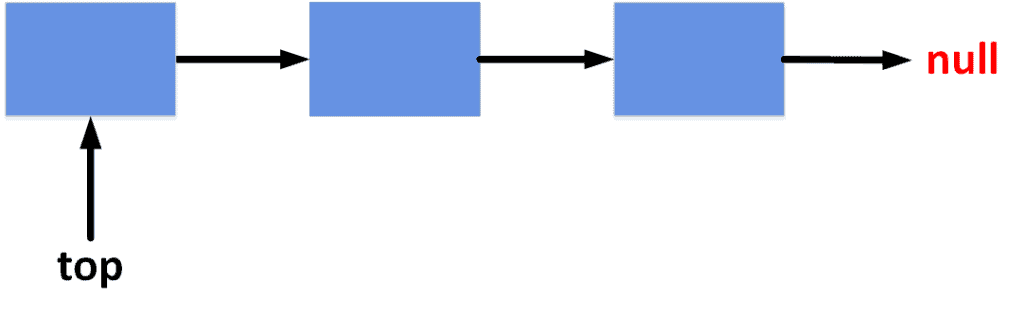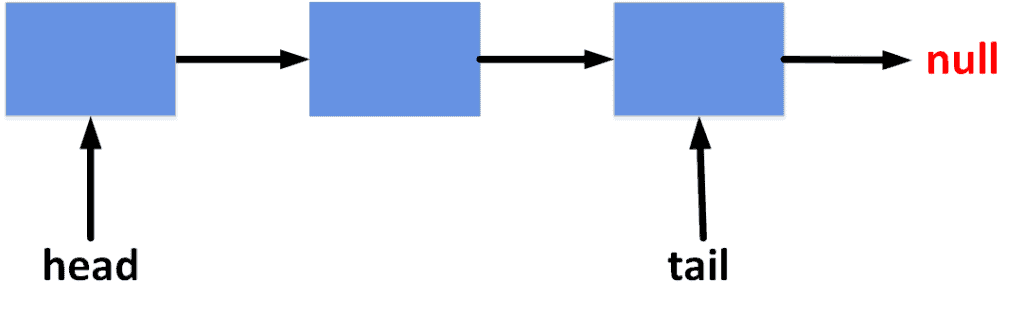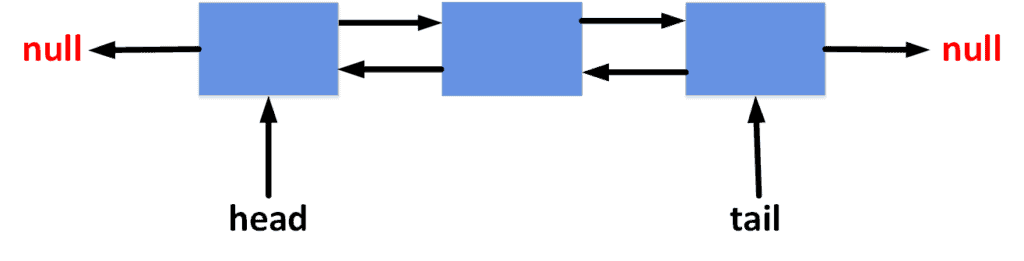## 1. Overview

In this tutorial, we’ll explain the complexities of operations on the main data structures like stacks, queues, deques, and sets. For each of them, we’ll shortly list the main operations and explain the complexity behind them.

## 2. Stacks

The stack data structure supports the operations of adding and retrieving elements in a LIFO order (Last In, First Out). This term refers to the fact that new elements are added to the beginning of the stack, and the first element to be retrieved is the last added element.

The complexity of these operations varies depending on the implementation. The most efficient way to implement a stack is using a simple linked list. Thus, we’ll discuss the complexity of each operation, assuming the stack is implemented using a linked list.

Take a look at the figure showing the general overview of a stack:The list is controlled by referencing the first element, usually the top.

Adding an element to a stack is usually called aoperation. The operation is simply done by adding a new element before theand changing the top element to become the newly added one.

The complexity of such an operation isbecause we only do constant timing operations.

### 2.2. Remove an Element

Removing an element from the top of the stack is called aoperation. It’s achieved by moving thereference one step forward to the next element. Thus, the time complexity of this operation is also.

### 2.3. Get an Element

In stacks, we usually want to know the value of the top element. This operation is simply done by retrieving the value of the element referenced by thepointer. Thus, it has a constant time complexity equal to.

### 2.4. Check Emptiness

When we want to check whether the stack contains any elements or not, we can check whether thereference points toor not. If so, the stack is empty. Otherwise, it has at least one element. This check can be implemented in antime complexity.

## 3. Queues

Queues support the operation of lining up elements one after the other. All new elements are added to the end of the queue, while the first element to be retrieved from the queue is the first added element. This is also known as FIFO (First In, First Out).

Similar to stacks, the most efficient way to implement queues is using linked lists. However, since adding and retrieving elements happen from two different ends of the list, we use two pointer references to hold the first and last elements. The following shape describes the general overview of a queue:We can call the reference to the first element the, while the reference to the last one is the. Note that, in a queue, each element points to the next one to make it easier to support adding and removing elements.

Similar to a stack, the operation of adding an element to a queue is called aoperation. It can be achieved by adding the new element after thereference and moving theto point to the newly added element. Since the operation is achieved by moving references with a constant time, the overall complexity is.

### 3.2. Remove an Element

As described, we remove elements from the beginning of the queue. This is done by moving thereference one step forward and deleting the element that was excluded from the queue. We can implement the operation intime complexity.

### 3.3. Get an Element

Since we are always interested in the element at the beginning of the queue, we need to return the value of the element that is pointed to by thereference. This operation has a time complexity of.

### 3.4. Check Emptiness

Checking whether a queue is empty is done by checking the value of either theorreferences. If they point to, then it means the queue is empty. Implementing this check is done withtime complexity.

## 4. Deques

Deques mix the behavior of stacks and queues. We can add elements to the beginning and end of a deque. Plus, we can retrieve elements from either end. To achieve this, we can implement deques using a double-linked list. Take a look at the following diagram to get an idea of the general structure of deques:We have two pointer references calledand, which allow us to add and delete elements from both ends.

As described, we can add elements to the beginning and end of a deque. These operations are usually calledand. We can implement them by adding an element before theor after theand moving the corresponding references.

Since it’s just moving pointer references, the overall complexity is.

### 4.2. Remove an Element

We can remove elements from both ends with operations calledand. This is done by moving thepointer one step forward or thepointer one step backward. Thus, the time complexity is.

### 4.3. Get an Element

We can get either the first or last element from a deque by getting the value of the element at the position of theorreferences. The time complexity for such operations is.

### 4.4. Check Emptiness

To check whether a deque is empty, it is enough to check whether theoris. If so, then the deque is empty. This can be done intime complexity.

## 5. Sets

Sets data structures are more complex than stacks, queues, and deques. We have two types of sets. Either it is an ordered set, which keeps the elements sorted, or a hash set, which hashes the elements to make it easier to find them. As a reminder, sets only allow each element to be added once.

Ordered sets are usually implemented with red-black trees to support the main operations in logarithmic time complexity. On the other hand, hash sets are implemented using the hash table data structure.

In the case of an ordered set, adding an element has a time complexity of, whereis the number of elements already in the set. This is because red-black trees have to keep the elements sorted.

Since hash sets rely on hash tables, the complexity is constant. In general, the complexity is close to.

### 5.2. Remove an Element

To remove an element from an ordered set, the red-black tree has to remain sorted. Thus, the complexity for ordered sets is, whereis the number of elements in the set. Hash sets, however, only need to hash the element to find and remove it. Therefore, the complexity for hash sets is.

### 5.3. Get an Element

To find an element in an ordered set, we needtime complexity, whereis the number of elements in the set. However, with hash sets, we can quickly find the element by hashing its value. Hence, the complexity for hash sets is.

### 5.4. Check Emptiness

Checking if the set is empty or not has a constant time complexity for both ordered sets and hash sets. The complexity in both cases is.

## 6. Comparison

The following table summarizes a comparison between the complexities in different data structures:The actual usage depends on the required behavior. Thus, defining which data structure to use is done by studying the use case and finding the best data structure to support the main operations efficiently.

## 6. Conclusion

In this article, we discussed the big-O notations for the complexities of main operations in stacks, queues, deques, and sets. We presented each of them separately. Then, we showed a summary comparison between all of them.